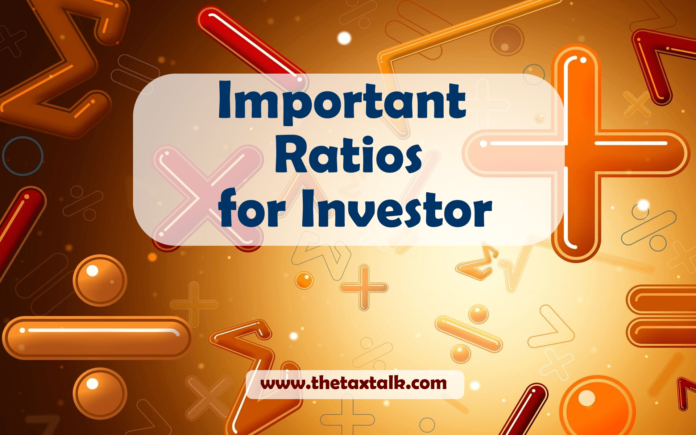# Important Ratios for Investor

0
151Price-to-Earnings Ratio (P/E)

P/E Ratio = Price per Share / Earnings Per Share

It is considered as one of the most important ratio from investor’s point of view.

Dividend Yield

Dividend Yield = Dividend per Share / Price per Share

Dividends are the main way companies return money to their shareholders. Dividend yield is used to compare different dividend-paying stocks. Some people prefer to invest in companies with a steady dividend, even if the dividend yield is low, while others prefer to invest in stocks with a high dividend yield.

Dividend Payout Ratio

Dividend Payout Ratio = Dividend / Net Income

The percentage of profits distributed as a dividend is called the dividend payout ratio. Each company sets its own dividend policy in the best interest of its shareholders.

Return on Equity (ROE)

Return on Equity = Net Income / Average Stockholder Equity

ROE tells you how good a company is at rewarding its shareholders for their investment. ROE higher is better.

Return on Assets (ROA)

Return on Assets = Net Income / Average Total Assets

A company buys assets in order to conduct its business. ROA tells you how good the company is at using its assets to make money.

Profit Margin

Profit Margin = Net Income / Sales

Profit margin calculates how much of a company’s total sales flow through to the bottom line. As you can probably tell, higher profits are better for shareholders, as is a high (and/or increasing) profit margin.

Price-to-Sales Ratio

Price-to-Sales Ratio = Price per Share / Annual Sales per Share

The P/S ratio is a great tool because sales figures are considered to be relatively reliable while other income statement items, like earnings, can be easily manipulated by using different accounting rules.

Current Ratio

Current Ratio = Current Assets / Current Liabilities

The current ratio measures a company’s ability to pay its short-term liabilities with its short-term assets. If the ratio is over 1.0, the firm has more short-term assets than short-term debts.

Quick Ratio

Quick Ratio = (Current Assets – Inventory) / Current Liabilities

The quick ratio backs out inventory because it assumes that selling inventory would take several weeks or months. The quick ratio only takes into account those assets that could be used to pay short-term debts today.

Debt to Equity Ratio

Debt-to-Equity Ratio = Total Liabilities / Total Shareholder Equity

The debt-to-equity ratio measures the relationship between the amount of capital that has been borrowed and the amount of capital contributed by shareholders .As a firm’s debt-to-equity ratio increases, it becomes more risky because if it becomes unable to meet its debt obligations.

Interest Coverage Ratio

Interest Coverage Ratio = EBIT / Interest Expense

Both EBIT and interest expense are found on the income statement. The interest coverage ratio, also known as times interest earned is a measure of how well a company can meet its interest payment obligations. If a company can’t make enough to make interest payments, it will be forced into bankruptcy.

Asset Turnover Ratio

Asset Turnover Ratio = Sales / Average Total Assets

Like return on assets (ROA), the asset turnover ratio tells you how good the company is at using its assets to make products to sell.